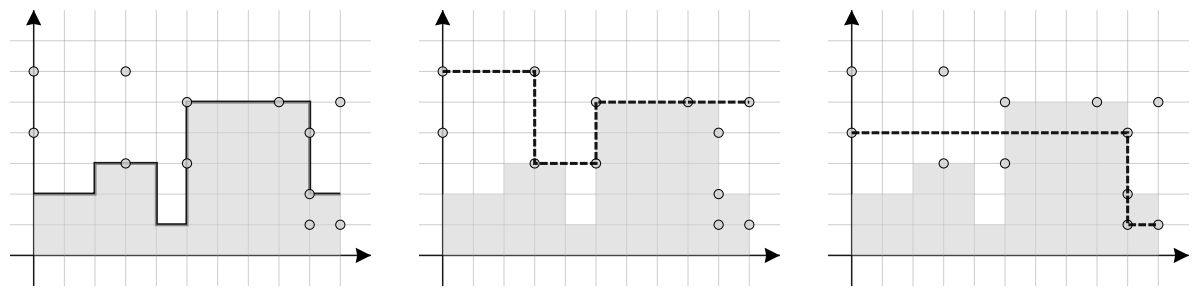시간 제한메모리 제한제출정답맞힌 사람정답 비율
1 초 256 MB33161445.161%

## 문제

A histogram is a graphical representation of a statistical distribution of data. In other words, it is a function that assigns a positive integer value to each number in the interval 0, 1, 2, . . . , W − 1. For this task, we describe a histogram with a series of points in the standard coordinate system which, sequentially, determine the top edge of the area that the histogram encloses.

More specifically, we define a histogram of width W with an even number of points in the form:

(x0, y1),(x1, y1),(x1, y2),(x2, y2),(x2, y3), . . . ,(xN/2−1, yN/2),(xN/2, yN/2).

Therefore, starting from the first point, for every pair of adjacent points it must hold that their y coordinates are equal and that their x coordinates are equal. Thus, the histogram begins and ends with a horizontal segment and in between horizontal and vertical segments alternate.

Additionally, the following holds for the shape of a histogram:

1. x0 = 0 - the histogram begins on the line x = 0.
2. xN/2 = W - the histogram ends on the line x = W.
3. xi < xi+1, for each i = 1, 2, . . . , N/2 − 1 - the histogram is defined from left to right and the length of each horizontal segment is at least one.
4. yi > 0 - the height of the histogram is always greater than zero.
5. yi ≠ yi+1 - the length of each vertical segment is at least one

For a histogram H, let $$y_H(x)$$ be the height of the histogram in the interval <x, x+1>. For example, the surface area which the histogram encloses can be calculated by formula $$\sum_{x=0}^{W-1}{y_H(x)}$$. If two histograms, H and H', are given, with equal width W (which can possibly be defined with a different number of points), then we can measure the difference between these two histograms in various ways. This measure of difference between two histograms is also called inaccuracy of histogram H’ with regard to histogram H. In this task, we examine two different ways of measuring differences between two histograms and we define them in the following way:

$\text{diffcount}(H^{\prime}, H) = \sum_{x=0}^{W-1}{ \text{diff}(y_H(x), y_{H^{\prime}}(x))} \text{ , where diff}(y_1,y_2) = 0 \text{ if }y_1 = y_2\text{, and }1 \text{else.} \\ \text{abserror}(H^{\prime}, H) = \sum_{x=0}^{W-1}{ |y_H(x), y_{H^{\prime}}(x)|}$

In other words, the first way measures the number of unit segments <x, x + 1>. in which the two histograms differ, whereas the second way is the sum of absolute values of differences on those individual unit segments.

Write a program that will, for a given histogram H, set of points S and way of measuring inaccuracy, find and output histogram H' which only uses points from the set S in its definition and has the least possible inaccuracy with regard to the given histogram H.Figure 1: Illustration of the histogram and the set S in both test cases given below and the solutions for the first and the second way of measuring inaccuracy.

The definition of histogram H' must comply with all aforementioned conditions (for example, there shouldn’t be two continuous horizontal segments in the definition of histogram H'), and each point in the definition must be one of the points from the set S.

## 입력

The first line of input contains integers N, M, G (2 ≤ N ≤ 100 000, 2 ≤ M ≤ 100 000, 1 ≤ G ≤ 2, N even), respectively: the total number of points in the definition of histogram H, number of points in set S and the number which determines what method of measuring the inaccuracy is used: 1 for diffcount and 2 for abserror.

Each of the following N lines contains integers X and Y (0 ≤ X ≤ 106, 1 ≤ Y ≤ 106) - coordinates of one point from the definition of histogram H. The histogram’s definition will comply to all the conditions from the task statement.

Each of the following M lines contains integers X and Y (0 ≤ X ≤ 106, 1 ≤ Y ≤ 106) - coordinates of one point from set S. All the points in the set S will be different from one another, but they can match with points from the definition of the histogram H.

## 출력

The first line of output must contain the least possible inaccuracy D. The second line of output must contain an even integer L - the total number of points in the definition of the required optimal histogram. In each of the following L lines, output two integers X and Y - coordinates of the point in the histogram’s definition.

The histogram’s definition must comply to all the conditions from the task.

## 예제 입력 1

10 12 1
0 2
2 2
2 3
4 3
4 1
5 1
5 5
9 5
9 2
10 2
0 4
0 6
3 3
3 6
9 4
9 1
5 3
5 5
10 5
9 2
10 1
8 5


## 예제 출력 1

5
6
0 6
3 6
3 3
5 3
5 5
10 5


## 예제 입력 2

10 12 2
0 2
2 2
2 3
4 3
4 1
5 1
5 5
9 5
9 2
10 2
0 4
0 6
3 3
3 6
9 4
9 1
5 3
5 5
10 5
9 2
10 1
8 5


## 예제 출력 2

14
4
0 4
9 4
9 1
10 1